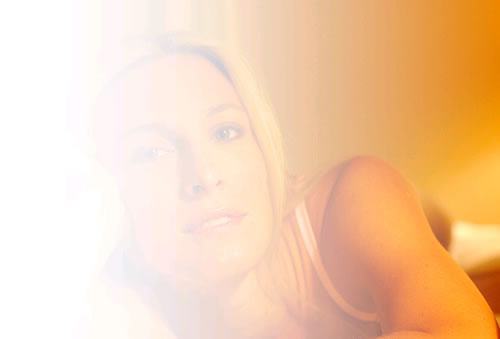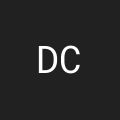By on
Tags:

I have added two functions to the ImageUtils.cfc ColdFusion image manipulation component. Building on top of the previously added function, CalculateGradient(), I have now added a function, CreateGradient(), that will create a gradient rectangle on its own canvas and DrawGradientRect(), which will draw a gradient rectangle on top of an existing image.

This method just creates the gradient canvas. The From and To colors are structs that contain Red, Green, Blue, and Alpha keys for the different color channels. The GradientDirection is the direction of the gradient on the canvas; the possible values for this are TopBottom, BottomTop, LeftRight, and RightLeft. The width and height constitute the dimensions of the gradient rectangle.

This method takes a given image and creates the gradient rectangle of the given dimensions (width / height) at the given coordinates (X / Y). The FromColor, ToColor, and GradientDirection are just used to call the CreateGradient() method so the same rules apply to the arguments.

Note: When creating a gradient, we have the option to use the Alpha channel. The Alpha channel goes from 255 which is totally NOT see-through, down to zero, which is totally transparent. Just realize that when you use ColdFusion's ImageSetDrawingTransparency() method, the numbers are in the opposite direction; meaning, a zero alpha which is see-through has a 100 transparency. This makes sense, but the conflicting values might be confusing.

Now that we see what the functions do, let's take a look at a practical example. Here, we are going to read in the image of the Subject and then draw a gradient rectangle over her canvas. Our gradient is going to stay white, but will fade from opaque to transparent:

``````<!---
Create to/from colors. In this case we just want to fade
the alpha channel, not the RGB channels so therefore, the
To/From colors are both white.
--->
<cfset objFromColor = HextoRGB( "##FFFFFF" ) />
<cfset objToColor = HextoRGB( "##FFFFFF" ) />

<!---
transparent which will have an alpha value of zero.
--->
<cfset objToColor.Alpha = 0 />

<!--- Read in the subject image. --->
<cfimage
source="./subject.jpg"
name="objImage"
/>

<!---
Apply the gradient rectangle. In this case, we want the
gradient to cover the entire image, so we are going to
paste in the 0,0 coordinate and use the entire width and
height of the image.
--->
objImage,
0,
0,
ImageGetWidth( objImage ),
ImageGetHeight( objImage ),
objFromColor,
objToColor,
"LeftRight"
) />

<!--- Draw image. --->
<cfimage
action="writetobrowser"
source="#objImage#"
/>
``````

Notice that after we convert our colors to RGB, we have to set the Alpha channel for our To color to be zero (fade to transparent). Running the above code, we get the following output:Not bad right? Can anyone guess where we go from here? (Think - image reflection???).

Here is the CreateGradient() ColdFusion image manipulation function that really powers this whole effect:

``````<cffunction
access="public"
returntype="any"
output="false"
hint="Creates a gradient rectangle to be used with other graphics.">

<!--- Define arguments. --->
<cfargument
name="FromColor"
type="struct"
required="true"
hint="The R,G,B,A struct for the start color of our gradient."
/>

<cfargument
name="ToColor"
type="struct"
required="true"
hint="The R,G,B,A struct for the end color of our gradient."
/>

<cfargument
type="string"
required="true"
hint="The direction in which to darw the gradient. Possible values are TopBottom, BottomTop, LeftRight, and RightLeft."
/>

<cfargument
name="Width"
type="numeric"
required="true"
hint="The width of the desired rectangle."
/>

<cfargument
name="Height"
type="numeric"
required="true"
hint="The height of the desired rectangle."
/>

<!--- Define the local scope. --->
<cfset var LOCAL = {} />

<!--- Make sure that we have a valid direciton. --->
<cfif NOT ListFind(
"TopBottom,BottomTop,LeftRight,RightLeft",
)>

<!--- Inavlid gardient, default to TopBottom. --->

</cfif>

<!---
Create a new transparent gradient. It is important
that it is transparent since the gradient utilizes
an alpha channel.
--->
"",
ARGUMENTS.Width,
ARGUMENTS.Height,
"argb",
""
) />

<!---
In order to figure out what steps that we need to
create, we need to figure out which direction the
--->

<!---
For vertical gradients, use the height to
define the number of steps.
--->
<cfcase value="TopBottom,BottomTop" delimiters=",">
<cfset LOCAL.StepCount = ARGUMENTS.Height />
</cfcase>

<!---
For horizontal gradients, use the width to
define the number of steps.
--->
<cfcase value="LeftRight,RightLeft" delimiters=",">
<cfset LOCAL.StepCount = ARGUMENTS.Width />
</cfcase>

</cfswitch>

<!---
Calculate the gradient using our From and To colors.
This will give us all the colors in the gradient.
--->
ARGUMENTS.FromColor,
ARGUMENTS.ToColor,
LOCAL.StepCount
) />

<!---
Now that we have our gradient steps, we can start to
apply our individual color steps to the blank canvas
in order to create the gradient rectangle.
--->

<!---
We don't want there to be too much fuziness, so turn
off antialiasing.
--->
<cfset ImageSetAntialiasing( LOCAL.Gradient, "off" ) />

<!--- Loop over the steps in the gradient. --->
<cfloop
index="LOCAL.StepIndex"
from="1"
to="#LOCAL.StepCount#"
step="1">

<!--- Set the current drawing color. --->
<cfset ImageSetDrawingColor(
(
LOCAL.GradientSteps[ LOCAL.StepIndex ].Red & "," &
LOCAL.GradientSteps[ LOCAL.StepIndex ].Green & "," &
)) />

<!---
Set the drawing transparency. When doing this,
we have to be careful as we are not setting the
opacity, which is actually the opposite value. An
alpha channel of 255 is totally opaque, but requires
a tranparency of zero.
--->
<cfset ImageSetDrawingTransparency(
(100 - (LOCAL.GradientSteps[ LOCAL.StepIndex ].Alpha / 255 * 100))
) />

<!---
When we actually draw the rectangle, we have to take
into account the direction of the gradient to figure
out where the individual step gradient will be applied.
--->
<cfcase value="TopBottom">

<cfset ImageDrawRect(
0,
(LOCAL.StepIndex - 1),
ARGUMENTS.Width,
1,
true
) />

</cfcase>
<cfcase value="BottomTop">

<cfset ImageDrawRect(
0,
(ARGUMENTS.Height - LOCAL.StepIndex),
ARGUMENTS.Width,
1,
true
) />

</cfcase>
<cfcase value="LeftRight">

<cfset ImageDrawRect(
(LOCAL.StepIndex - 1),
0,
1,
ARGUMENTS.Height,
true
) />

</cfcase>
<cfcase value="RightLeft">

<cfset ImageDrawRect(
(ARGUMENTS.Width - LOCAL.StepIndex),
0,
1,
ARGUMENTS.Height,
true
) />

</cfcase>
</cfswitch>

</cfloop>

</cffunction>
``````

Then, we have the DrawGradientRect() ColdFusion image manipulation function. As you can see below, this function really just turns around and calls the CreateGradient() method:

``````<cffunction
access="public"
returntype="any"
output="false"
hint="Takes an image and draws the given gradient rectangle on it.">

<!--- Define arguments. --->
<cfargument
name="Image"
type="any"
required="true"
hint="The ColdFusion image object onto which we are drawing the gradient."
/>

<cfargument
name="X"
type="numeric"
required="true"
hint="The X coordinate at which to start drawing the rectangle."
/>

<cfargument
name="Y"
type="numeric"
required="true"
hint="The Y coordinate at which to start drawing the rectangle."
/>

<cfargument
name="Width"
type="numeric"
required="true"
hint="The width of the desired rectangle."
/>

<cfargument
name="Height"
type="numeric"
required="true"
hint="The height of the desired rectangle."
/>

<cfargument
name="FromColor"
type="struct"
required="true"
hint="The R,G,B,A struct for the start color of our gradient."
/>

<cfargument
name="ToColor"
type="struct"
required="true"
hint="The R,G,B,A struct for the end color of our gradient."
/>

<cfargument
type="string"
required="true"
hint="The direction in which to darw the gradient. Possible values are TopBottom, BottomTop, LeftRight, and RightLeft."
/>

<!--- Define the local scope. --->
<cfset var LOCAL = {} />

<!--- Create the gradient rectangle. --->
ARGUMENTS.FromColor,
ARGUMENTS.ToColor,
ARGUMENTS.Width,
ARGUMENTS.Height
) />

<!--- Paste the gradient onto the image. --->
<cfset ImagePaste(
ARGUMENTS.Image,
ARGUMENTS.X,
ARGUMENTS.Y
) />

<!--- Return the updated image. --->
<cfreturn ARGUMENTS.Image />
</cffunction>
``````

There's a few reasons that we are splitting this functionality up into two different methods. For starters, it saves you from having to call the CreateGradient() method and translate the logic. But mostly, we want to create a gradient method that is independent of the target image in order to allow it to be reused by various other methods. As I have hinted above, we can now start to use this feature and even the DrawGradientRect() method to create things like image reflection effects. The more we can split things up into smaller, more cohesive pieces of functionality, the more we can start to reuse them.

Want to use code from this post? Check out the license.Hey Ben,

Was there a particular reason that you have the "passthrough" parameters for DrawGradientRect in a different order than they are in CreateGradient? It seems to me to make the order of parameters the same would make them easier to remember (as well as potentially copy and paste when moving from a CreateGradient to DrawGradientRect as your needs change). Unless of course you're using an application that gives you the code hints for CFC methods. (Speaking of that...Ben, write me and I might be able to share a a little toy to play with in Dreamweaver)

Also, it might be good to add a Gradient object/struct to the mix, and that way you can pass it around into these types of functions, especially if you're going to do "reflector-ization" on multiple images as some sort of batch processing.@Danilo,

It's funny that you bring that up because I definitely had them in the same order on the first run and then went back and changed them. I think part of me wanted to emphasize the "gradient" part of the CreateGradient() method. I almost wanted to *stop* the copy-paste mentality of the arguments - I wanted people to think about what the context of the method was, not just that one turns around and calls another.

I am, however, not really sold one way or the other. And, actually, now that I think of it, I believe that the rest of ColdFusion's image manipulation functions have the coordinates and dimensions first. So, I guess in that respect, it would be more consistent to have width / height of the gradient first and then the colors.

As far as the gradient struct, I am not 100% sure what you are saying. I think you mean that we would have to recalculate the gradient for each image, and if we are doing a lot of images in a row, that could be a lot of work?? If that is what you mean, I could certainly see the ability to call the CalculateGradient() method to return the array, and then some sort of ApplyGradient() method that uses the same array of color indexes to an image.Ben,

My initial thought was that you could pass the DrawGradientRect a Gradient struct with the properties that are needed by CreateGradient DrawGradientRect, but then I was also thinking about the calculated set of color values that could then be used multiple times. As I've not looked at the implementation of these functions, it could be useful whether they are simple pixel color values for the entire rectangle, or if an actual gradient is specified without needing to set each pixel individually. More useful if you have to set each pixel's color value within the rectangle.

So anyway, it could be as simple as a struct that has just the properties needed, or as complex as the fully calculated pixel values for the gradiented (?) rectangle.

So now that you have linear gradients, how about other shapes as well? If you're going down that route, then you may want to have a gradient struct with all the parameters and then pass in a type attribute to the CreateGradient so that it can handle linear, circular, and other types of gradients (whatever they may be).